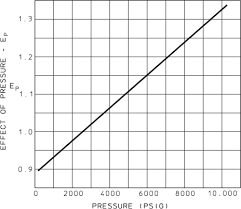## How to Calculate and Solve for Bulk Modulus of Elasticity (stress) | Rock MechanicsThe image above represents bulk modulus of elasticity (stress).

To compute for bulk modulus elasticity (stress), two essential parameters are needed and these parameters are Average Horizontal Stress (hav) and Vertical Stress (σv).

The formula for calculating the bulk modulus elasticity (stress):

k = hav / σv

Where;

k = Bulk Modulus of Elasticity (Stress)
hav = Average Horizontal Stress
σv = Vertical Stress

Let’s solve an example;
Given that the average horizontal stress is 15 and the vertical stress is 34. Find the bulk modulus of elasticity (stress)?

This implies that;

hav = Average Horizontal Stress = 15
σv = Vertical Stress = 34

k = hav / σv
k = 15 / 34
k = 0.44

Therefore, the bulk modulus of elasticity (stress) is 0.44.

Calculating the Average Horizontal Stress when the Bulk Modulus Elasticity (stress) and the Vertical Stress is Given.

hav = k x σv

Where;

hav = Average Horizontal Stress
k = Bulk Modulus of Elasticity (Stress)
σv = Vertical Stress

Let’s solve an example;
Given that the bulk modulus of elasticity (stress) is 25 and the vertical stress is 8. Find the average horizontal stress?

This implies that;

k = Bulk Modulus of Elasticity (Stress) = 25
σv = Vertical Stress = 8

hav = k x σv
hav = 25 x 8
hav = 200

Therefore, the average horizontal stress is 200.